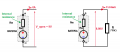# Output impedance of source follower

#### ray242

Joined Nov 27, 2016
44
Hi, I've been learning about JFET and I came across the source follower. My book gives me two ways for calculating the output impedance (as shown in the picture, by shorting the input etc.) but I don't understand why it is feasible. Could someone explains it to me in more detail?

#### Jony130

Joined Feb 17, 2009
5,180
Hi, Have you ever tried to measure in real life an Internal resistance of a voltage source (battery)?
You only have a one voltmeter and one given load resistance ?

So first what we do is to measure a "open" source voltage ( Vsig = 9V ) next we connect a given load resistance (750Ω) to the battery terminals and measure the output voltage Vout = 8.88V.And from there we have to find the battery Internal resistance Rw. Do you know how to do this ?

In your case we have almost exactly the same situation. Except the fact that now we do not know the value of a Load resistance, but we know Vsig and Rw (Internal resistance) values.

Last edited:
•ray242

#### MrAl

Joined Jun 17, 2014
7,757
Hi, I've been learning about JFET and I came across the source follower. My book gives me two ways for calculating the output impedance (as shown in the picture, by shorting the input etc.) but I don't understand why it is feasible. Could someone explains it to me in more detail?

Hi,

The method in part A is very basic. If you know how to measure input impedance then you know how to measure output impedance as you just pretend the output is the input.
However, that may be impractical for some circuits because you have to make sure you dont upset the biasing of the input or output of the circuit so they give you part B for an AC amplifier.

If you apply a reasonable voltage you measure the current and then calculate the resistance using Ohm's Law:
R=V/I

Sometimes a current source is used instead of a voltage source, and then you just measure the voltage and again use Ohm's Law:
R=V/I

•ray242

#### DickCappels

Joined Aug 21, 2008
6,533

#### MrAl

Joined Jun 17, 2014
7,757
Those are methods of measuring the output impedance.

To calculate it, see the "Characteristics" section of https://en.wikipedia.org/wiki/Common_drain

Hi there Dick,

Yes the wording makes it sound like we are 'measuring' but really this method applies when calculating also. That's because in theory we can treat the circuit output as an input just like we can when doing an actual real life measurement, so in a theoretical calculation we can apply a voltage or current source just like we do when doing an actual measurement. It is still good to point that out thoughYes there are others ways of doing it too.

•ray242